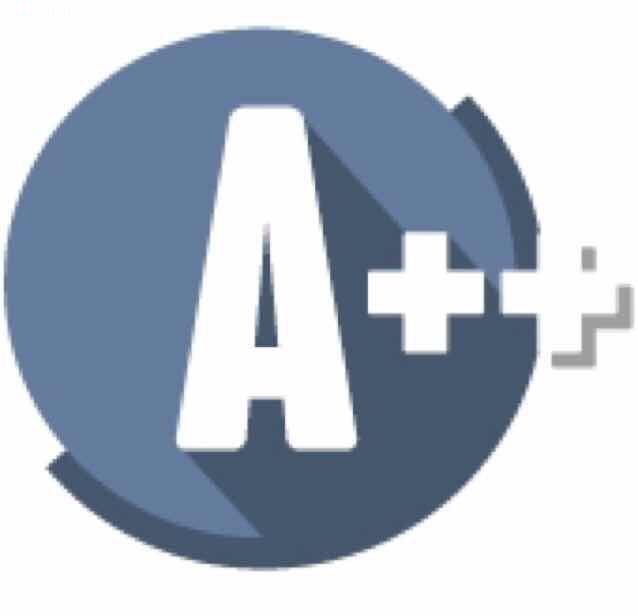## Interviews

#### Combination Sum Of CoinsGiven many coins of 3 different face values, print the combination sums of the coins up to 1000. Must be printed in order.

eg: coins(10, 15, 55)
print:
10
15
20
25
30
.
.
.
1000

Solution:
``````public void printSums(int c1, int c2, int c3) {

Set sums = new HashSet<>();

for(int sum = 1; sum <= 1000; sum++) {

if(sums.contains(sum - c1) || sums.contains(sum - c2) || sums.contains(sum - c3)) {
System.out.println(sum);
}
}
}
``````